In :
import numpy as np
import matplotlib.pyplot as plt
from sequentia.preprocessing import *

seed = 1
np.random.seed(seed)
rng = np.random.RandomState(seed)


# Preprocessing¶

This tutorial notebook describes some of the preprocessing methods offered by Sequentia.

## Preprocessing methods¶

Before we can look at the different preprocessing methods, we have to create a multivariate observation sequence, F, that we can use:

In :
# Specify an input domain for the functions
xs = np.arange(-4., 4., 0.025)

# Create two lambdas that define the features of the observation sequence
f1 = lambda x: (np.sin(x) - np.sin(np.exp(-0.7 * x)))
f2 = lambda x: (np.exp(np.cos(2*x)) * np.cos(x - 5))
# Auxilliary function for downsampling the domain (for plotting)
d = lambda x, n: Downsample(factor=n, method='decimate')(x)

# Compute function values evaluated on the provided domain (xs)
F1, F2 = f1(xs), f2(xs)
# Combine F1 and F2 to form the features for the multivariate sequence F
F = np.vstack((F1, F2)).T

plt.figure(figsize=(20, 5))
plt.title('Multivariate ($D=2$) observation sequence F composed of features F1 and F2', fontsize=16)
plt.plot(xs, F)
plt.show()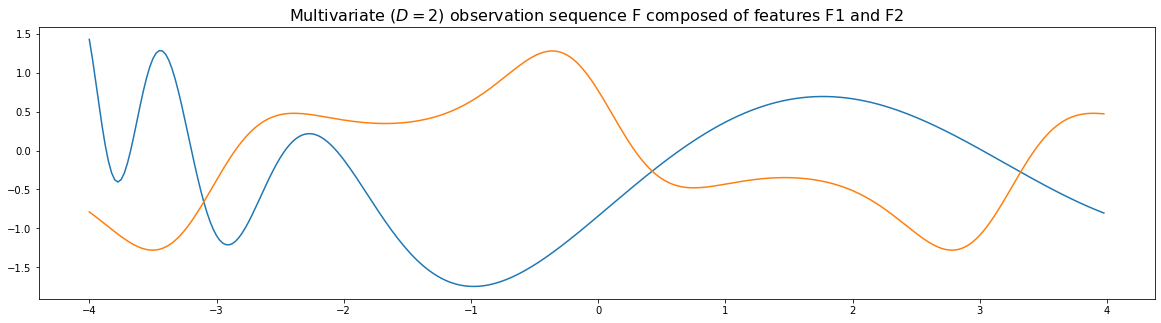### Downsampling¶

Reduces the number of frames in an observation sequence according to a specified downsample factor and one of two methods: averaging (mean) and decimation.

As an example, using a downsample factor of $n=5$ (and the decimation method – which is the default), we can significantly reduce the number of frames in the observation sequence:

In :
print('Original shape: {}'.format(F.shape))
print('Downsampled shape (n=5): {}'.format(Downsample(factor=5)(F).shape))

Original shape: (320, 2)
Downsampled shape (n=5): (64, 2)


#### Mean downsampling¶

With a downsample factor of $n$, mean downsampling replaces every group of $n$ consequent observations with a new observation given by mean of those observations – reducing the number of frames by approximately $\frac{1}{n}$.

The following plots allow us to see the effects of different downsample factors on the mean downsampling method:

In :
fig, axs = plt.subplots(2, 2, figsize=(15, 7), sharey=True)
fig.suptitle("Downsampling with the 'mean' method", fontsize=16)

axs[0, 0].set_title('Original sequence ({} frames)'.format(len(F)))
axs[0, 0].plot(xs, F)

down = Downsample(factor=5, method='mean')(F)
axs[0, 1].set_title('Downsample factor $n=5$ ({} frames)'.format(len(down)))
axs[0, 1].plot(d(xs, n=5), down, '--')

down = Downsample(factor=15, method='mean')(F)
axs[1, 0].set_title('Downsample factor $n=15$ ({} frames)'.format(len(down)))
axs[1, 0].plot(d(xs, n=15), down, '--')

down = Downsample(factor=25, method='mean')(F)
axs[1, 1].set_title('Downsample factor $n=25$ ({} frames)'.format(len(down)))
axs[1, 1].plot(d(xs, n=25), down, '--')

plt.tight_layout()
plt.show()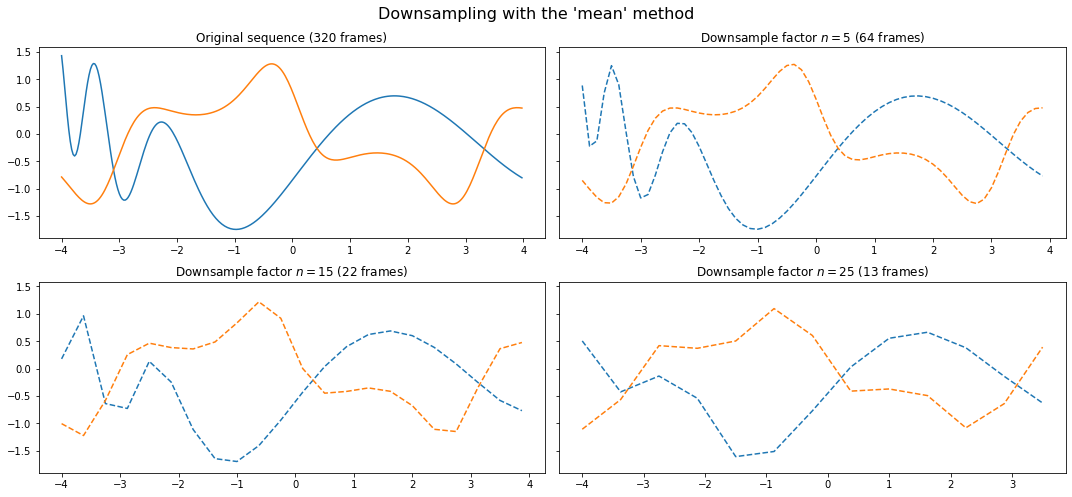Observe that even with just 64 frames, we can still capture most of the important information about the observation sequence – while making the sequence five times smaller!

This is often the case with observation sequences that are recorded using very high sample rates.

#### Decimation¶

With a downsample factor $n$, decimation keeps every $n^\text{th}$ observation and removes (decimates) the subsequent $n-1$ observations.

Once again, we can visualize the effects of the downsample factor by plotting the downsampled sequences.

In :
fig, axs = plt.subplots(2, 2, figsize=(15, 7), sharey=True)
fig.suptitle("Downsampling with the 'decimate' method", fontsize=16)

axs[0, 0].set_title('Original sequence ({} frames)'.format(len(F)))
axs[0, 0].plot(xs, F)

down = Downsample(factor=5, method='decimate')(F)
axs[0, 1].set_title('Downsample factor $n=5$ ({} frames)'.format(len(down)))
axs[0, 1].plot(d(xs, n=5), down, '--')

down = Downsample(factor=15, method='decimate')(F)
axs[1, 0].set_title('Downsample factor $n=15$ ({} frames)'.format(len(down)))
axs[1, 0].plot(d(xs, n=15), down, '--')

down = Downsample(factor=25, method='decimate')(F)
axs[1, 1].set_title('Downsample factor $n=25$ ({} frames)'.format(len(down)))
axs[1, 1].plot(d(xs, n=25), down, '--')

plt.tight_layout()
plt.show()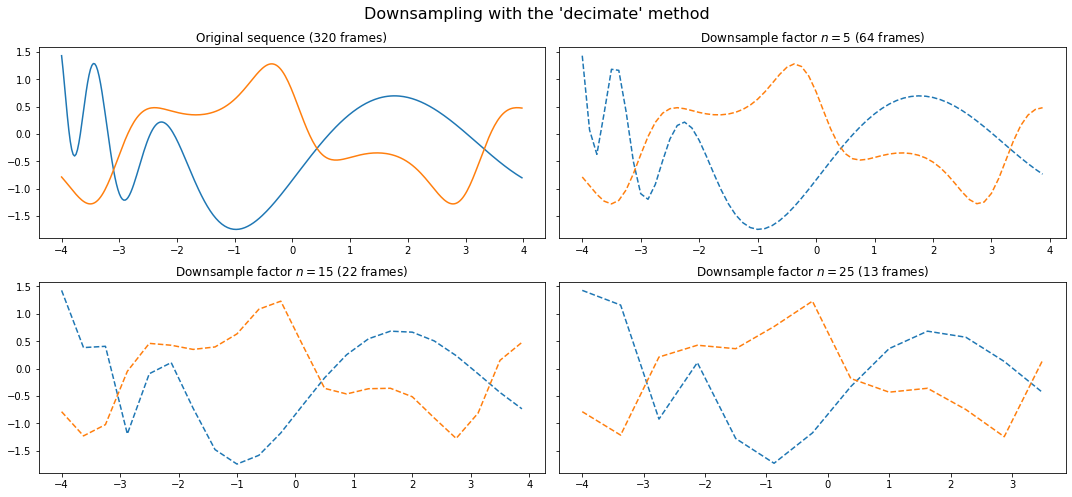From these plots, the differences between downsampling through decimation and averaging aren't immediately obvious.

Lets look at a comparison of the two methods using a downsample factor of $n=15$:

In :
fig, axs = plt.subplots(1, 2, figsize=(15, 5), sharey=True)
axs.set_title('Original sequence', fontsize=16)
axs.plot(xs, F1, label='F1')
axs.plot(xs, F2, label='F2')
axs.legend(fontsize=12)

axs.set_title('Downsampled sequence ($n=15$)', fontsize=16)
axs.plot(d(xs, n=15), Downsample(factor=15, method='mean')(F1), 'C0:', label='F1 (average)')
axs.plot(d(xs, n=15), Downsample(factor=15, method='decimate')(F1), 'C0--', label='F1 (decimate)')
axs.plot(d(xs, n=15), Downsample(factor=15, method='mean')(F2), 'C1:', label='F2 (average)')
axs.plot(d(xs, n=15), Downsample(factor=15, method='decimate')(F2), 'C1--', label='F2 (decimate)')
axs.legend(fontsize=12)

plt.tight_layout()
plt.show()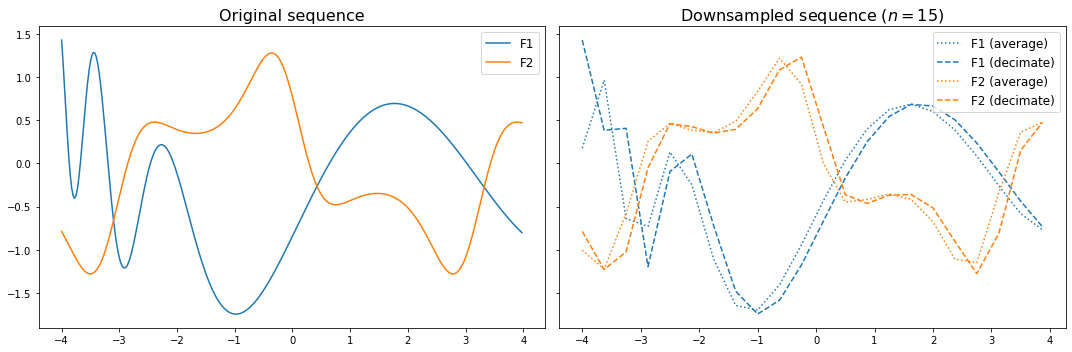Once again, it might not be straightforward to choose between the two methods. The best approach would probably be to choose the method that minimizes validation error.

### Zero trimming¶

Removes zero-observations from an observation sequence.

Many datasets consisting of sequential data often pad observation sequences with zeros in order to ensure that the machine learning algorithms receive sequences of equal length. Although this comes with the advantage of being able to represent the sequences in a matrix, the added zeros may affect the performance of the machine learning algorithms.

As the algorithms implemented by Sequentia focus on supporting variable-length sequences out of the box, zero padding is not necessary, and can be removed with this method.

Note: This preprocessing method does not only remove trailing zeros from the start or end of a sequence, but will also remove any zero-observations that occur anywhere in the sequence.

It is clear from the description what zero trimming does, but lets look at an example:

In :
fig, axs = plt.subplots(1, 2, figsize=(15, 5), sharey=True)

zeros = np.zeros((30, 2))
padded_F = np.vstack((zeros, F, zeros, zeros, zeros))

axs.set_title('Zero-trimmed sequence', fontsize=16)

plt.tight_layout()
plt.show()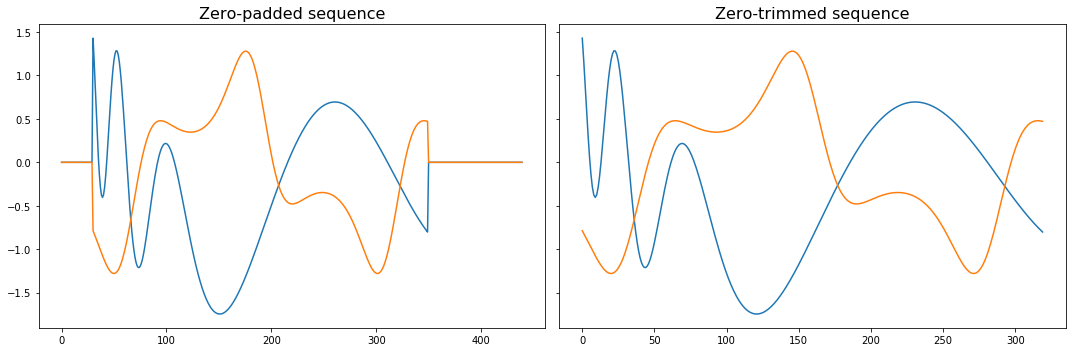### Centering¶

Centers an observation sequence by either:

• (default) centering each feature or dimension independently for each observation, so that they all have zero mean,
• centering each feature or dimension collectively (using a single mean for all observation sequences).

As a simple check on F:

In :
np.mean(Center()(F), axis=0)

Out:
array([-6.66133815e-17, -4.44089210e-17])

To see the effects of centering, we can simply plot the centered observation sequence:

In :
plt.figure(figsize=(20, 5))
plt.title('Observation sequence centering', fontsize=16)
plt.plot(xs, F1, label='F1')
plt.plot(xs, Center()(F1), 'C0--', label='F1 (centered)')
plt.plot(xs, F2, label='F2')
plt.plot(xs, Center()(F2), 'C1--', label='F2 (centered)')
plt.legend(fontsize=12)
plt.show()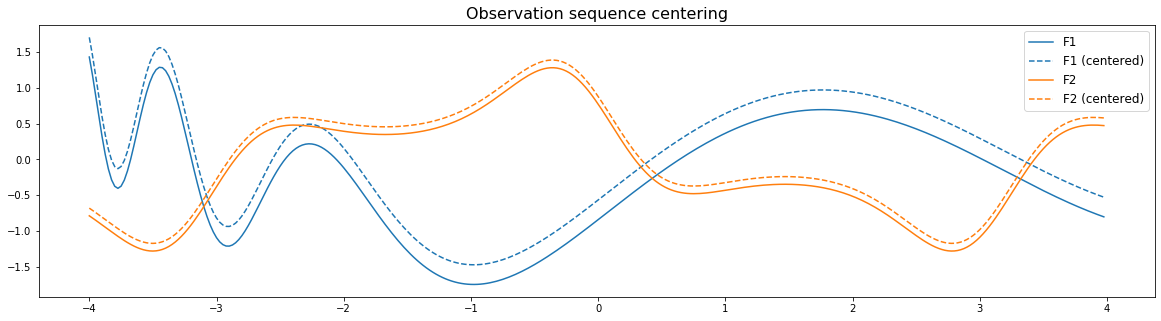### Standardizing¶

Standardizes an observation sequence by either:

• (default) transforming each feature or dimension individually for each observation sequence so that they each have zero mean and unit variance,
• transforming each feature or dimension collectively (using a single mean and standard deviation).

As a simple check on F:

In :
print('Mean: {}'.format(Standardize()(F).mean(axis=0)))
print('Deviation: {}'.format(Standardize()(F).std(axis=0)))

Mean: [ 0.00000000e+00 -2.22044605e-17]
Deviation: [1. 1.]


To see the effects of standardizing, we can simply plot the standardized observation sequence:

In :
plt.figure(figsize=(20, 5))
plt.title('Observation sequence standardizing', fontsize=16)
plt.plot(xs, F1, label='F1')
plt.plot(xs, Standardize()(F1), 'C0:', label='F1 (standardized)')
plt.plot(xs, F2, label='F2')
plt.plot(xs, Standardize()(F2), 'C1:', label='F2 (standardized)')
plt.legend(fontsize=12)
plt.show()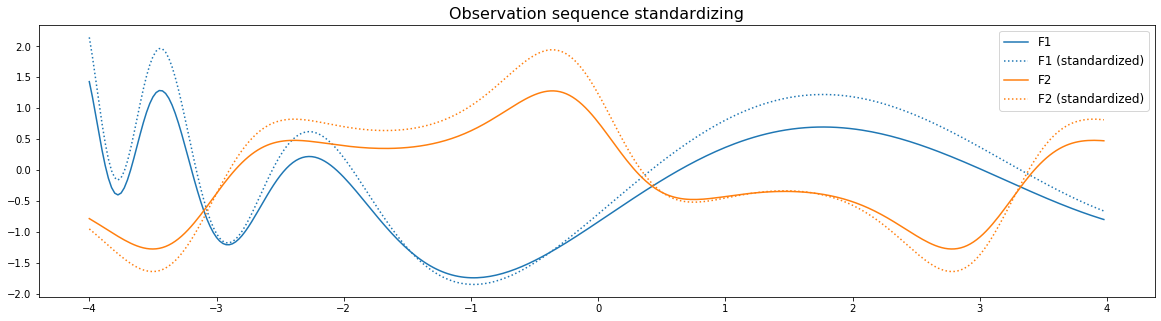### Filtering¶

Removes some unwanted components (such as noise) from an observation sequence according to some window size and one of two methods: median and mean filtering.

To demonstrate and visualize filtering methods, we need to add some noise to the original observation sequence.

In :
# Add noise to the original observation sequence
noise = 0.2 * rng.normal(0, 1, F.shape)
F_noisy = F + noise


#### Median filtering¶

With a window size of $n$, median filtering replaces every observation $\mathbf{o}^{(t)}$ with the median of the window of observations of size $n$ containing $\mathbf{o}^{(t)}$ in its centre:

$$\mathbf{o}^{(t)\prime}=\mathrm{med}\underbrace{\left[\ldots, \mathbf{o}^{(t-1)}, \mathbf{o}^{(t)}, \mathbf{o}^{(t+1)}, \ldots\right]}_n$$

The following plots allow us to see the effects of different window sizes on the median downsampling method:

In :
fig, axs = plt.subplots(2, 2, figsize=(15, 7), sharey=True)
fig.suptitle("Filtering with the 'median' method", fontsize=16)

axs[0, 0].set_title('Original sequence ({} frames)'.format(len(F)))
axs[0, 0].plot(F)

axs[0, 1].set_title('Original sequence with noise ({} frames)'.format(len(F_noisy)))
axs[0, 1].plot(F_noisy)

filtered = Filter(window_size=5, method='median')(F_noisy)
axs[1, 0].set_title('Filtered noisy sequence with window size $n=5$ ({} frames)'.format(len(filtered)))
axs[1, 0].plot(filtered, '--')

filtered = Filter(window_size=20, method='median')(F_noisy)
axs[1, 1].set_title('Filtered noisy sequence with window size $n=20$ ({} frames)'.format(len(filtered)))
axs[1, 1].plot(filtered, '--')

plt.tight_layout()
plt.show()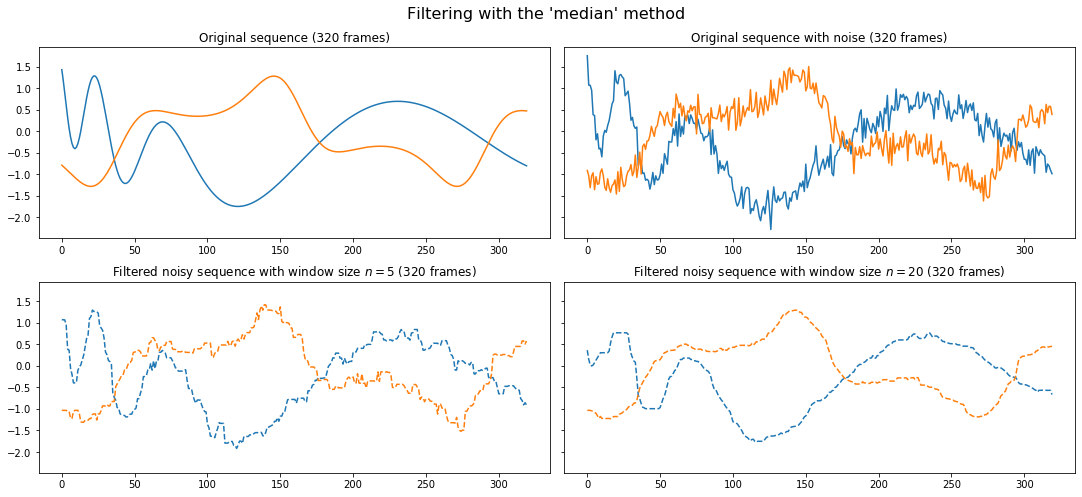The median filter does a good job at reducing the noise! With generally a smoother function as the window size increases.

#### Mean filtering¶

With a window size of $n$, mean filtering replaces every observation $\mathbf{o}^{(t)}$ with the mean of the window of observations of size $n$ containing $\mathbf{o}^{(t)}$ in its centre:

$$\mathbf{o}^{(t)\prime}=\mathrm{mean}\underbrace{\left[\ldots, \mathbf{o}^{(t-1)}, \mathbf{o}^{(t)}, \mathbf{o}^{(t+1)}, \ldots\right]}_n$$

The following plots allow us to see the effects of different window sizes on the mean downsampling method:

In :
fig, axs = plt.subplots(2, 2, figsize=(15, 7), sharey=True)
fig.suptitle("Filtering with the 'mean' method", fontsize=16)

axs[0, 0].set_title('Original sequence ({} frames)'.format(len(F)))
axs[0, 0].plot(F)

axs[0, 1].set_title('Original sequence with noise ({} frames)'.format(len(F_noisy)))
axs[0, 1].plot(F_noisy)

filtered = Filter(window_size=5, method='mean')(F_noisy)
axs[1, 0].set_title('Filtered noisy sequence with window size $n=5$ ({} frames)'.format(len(filtered)))
axs[1, 0].plot(filtered, '--')

filtered = Filter(window_size=20, method='mean')(F_noisy)
axs[1, 1].set_title('Filtered noisy sequence with window size $n=20$ ({} frames)'.format(len(filtered)))
axs[1, 1].plot(filtered, '--')

plt.tight_layout()
plt.show()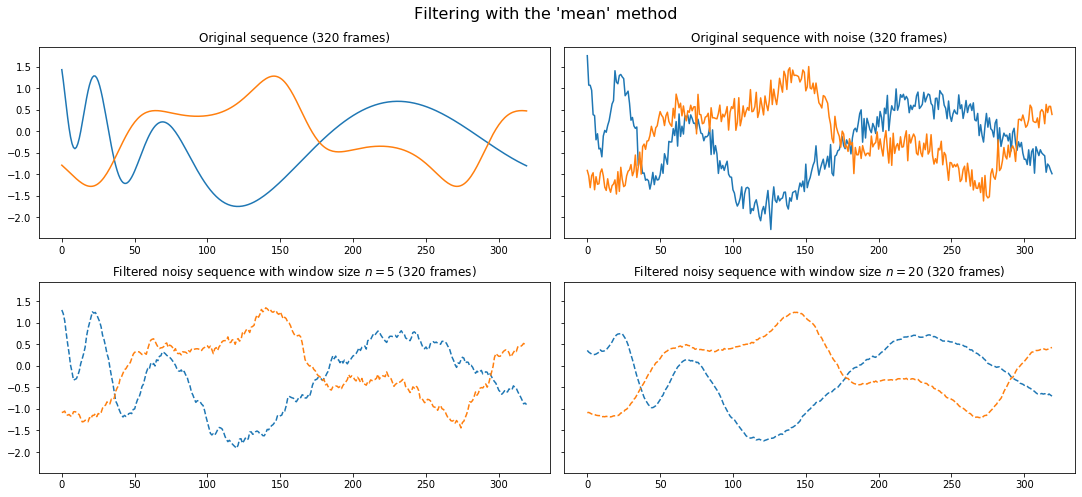Similarly to the downsampling methods, it often isn't clear which of the filtering methods will perform best.

## Accepted input formats¶

Just as with the fit() and predict() functions on the Sequentia classifiers, each of the preprocessing functions accepts:

• numpy.ndarray: A single multivariate observation sequence
• list(numpy.ndarray): Multiple multivariate observation sequences

Note: The observation sequences must strictly have a second dimension. If using one-dimensional observation sequences, these must be reshaped from (T,) to (T, 1), where T is the number of frames.

Nothing changes about how you call the preprocessing functions:

In :
# Single multivariate observation sequences
down = Downsample(factor=5, method='mean')(F)
down.shape

Out:
(64, 2)
In :
# Multiple multivariate observation sequences
Fs = [F]*5
down = Downsample(factor=5, method='mean')(Fs)
[f.shape for f in down]

Out:
[(64, 2), (64, 2), (64, 2), (64, 2), (64, 2)]

## Combining preprocessing methods¶

The Preprocess class can be used to chain preprocessing method calls:

In :
pre = Preprocess([
TrimZeros(),
Center(),
Standardize(),
Filter(window_size=10, method='median'),
Downsample(factor=15, method='decimate')
])

# Summarize the preprocessing steps
pre.summary()

                   Preprocessing summary:
============================================================
1. TrimZeros
Remove zero-observations
------------------------------------------------------------
2. Center
Centering around mean (zero mean) (independent)
------------------------------------------------------------
3. Standardize
Standard scaling (zero mean, unit variance) (independent)
------------------------------------------------------------
4. Filter
Median filtering with window-size 10
------------------------------------------------------------
5. Downsample
Decimation downsampling with factor 15
============================================================

In :
F_pre = pre.transform(Fs)
# Can also call as pre(Fs)